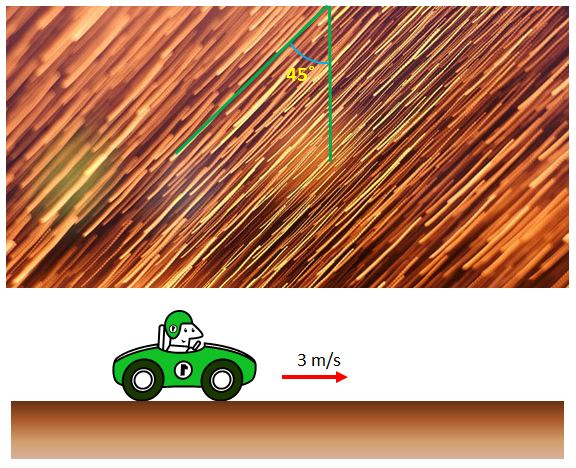# Rain DropThe above is a depiction of rain drops falling on a car being driven at a speed of $3$ m/s. If the angle of the rain drops on the car window is exactly $45$°, then what is the speed of the rain drops from a stopped observer's position? (Assume that the speed of the rain drops is constant and raindrops are falling straight down.)

×